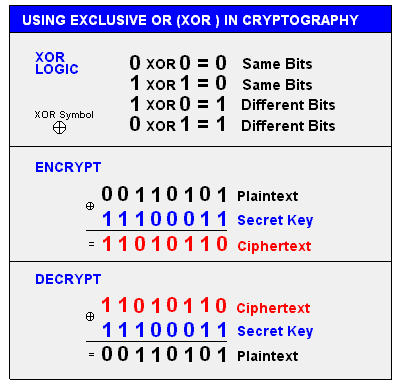# xor

(redirected from XORs)
Also found in: Dictionary.

## XOR

[eks′ȯr]
(computer science)

## XOR

(eXclusive OR) A Boolean logic operation that is widely used in cryptography as well as in generating parity bits for error checking and fault tolerance. XOR compares two input bits and generates one output bit. The logic is simple. If the bits are the same, the result is 0. If the bits are different, the result is 1.

Several Symbols
Various symbols are used to designate the XOR operation including a + sign inside a circle, an underlined "V" and the caret (^). See cryptography, RAID and or.

XOR in CryptographyThis example uses XOR and the same "secret key" to encrypt and decrypt. Although XOR logic may be used, secret key algorithms are much more sophisticated than this. See encryption algorithm.
References in periodicals archive ?
The accumulator of partial products, implemented by XOR gates in case of being sequential are fed back using flip-flop memory storage devices.
The proposed scheme, called Combiner, uses bitwise exclusive disjunction (XOR) for the manipulation of data within packets from different data channels.
It consists of two logic elements (LE), one LUT2-1, one XOR, and three multiplexers.
To communicate new group key [P.sub.K'] to nodes S0, ..., S3, CH computes the hash of key [K.sub.0-3] i.e., G([K.sub.0-3]) and XORs this with new group key PK' which yields [K.sup.S0, ..., S3] [left arrow] (G([K.sub.0-3])) XOR ([P.sub.K']).
* Primary controller XORs results (from step above) with new write data
A proper combination of simple operations such as XOR (exclusive or addition modulo 2, sometimes written as ??), substitution, and permutation, produces a cryptosystem whose strength is greater than the sum of its parts.
Linear network coding and exclusive or (XOR) are two major techniques in network coding.
The exclusive or (XOR) circuit acts as the OR element for events.
The symbol [direct sum] denotes the bitwise XOR, the bitwise complement AND the bitwise AND operation.
The four results are exclusive or'ed (XOR'ed) together.
Intermediate nodes first schedule a packet for sending then select sub set of incoming packets and apply XOR function to code with scheduled packet such that receiver can decode it.
The number of XORed source packets is defined as the code degree and it is sampled from the degree distribution function.

Site: Follow: Share:
Open / Close